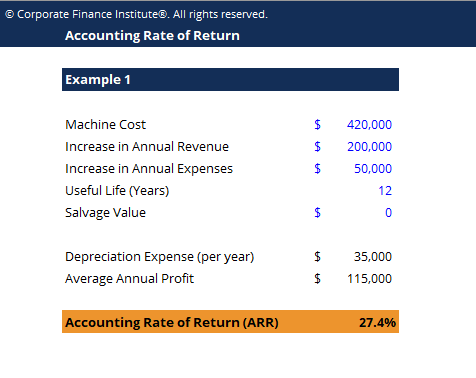# Accounting Rate of Return Template

## Accounting Rate of Return Template

This accounting rate of return template will demonstrate two examples of ARR calculation.

Below is a screenshot of the accounting rate of return template:### Download the Free Template

Enter your name and email in the form below and download the free template now!

### Accounting Rate of Return Template

Download the free Excel template now to advance your finance knowledge!

Accounting Rate of Return (ARR) is the average net income an asset is expected to generate divided by its average capital cost, expressed as an annual percentage. The ARR is a formula used to make capital budgeting decisions. These typically include situations where companies are deciding on whether or not to proceed with a specific investment (a project, an acquisition, etc.) based on the future net earnings expected compared to the capital cost.

The formula for ARR is: ARR = average annual profit / average investment

Where,

Average investment = (book value at year 1+ book value at end of useful life) / 2

Average annual profit = total profit over investment period/number of years

If the ARR is equal to 5%, this means that the project is expected to earn five cents for every dollar invested per year.

In terms of decision making, if the ARR is equal to or greater than the required rate of return, the project is acceptable because the company will earn at least the required rate of return.

If the ARR is less than the required rate of return, the project should be rejected. Therefore, the higher the ARR, the more profitable the investment.

### More Free Templates

For more resources, check out our business templates library to download numerous free Excel modeling, PowerPoint presentation and Word document templates.

• Excel Modeling Templates
• PowerPoint Presentation Templates
• Transaction Document Templates

### Financial Analyst Certification

Become a certified Financial Modeling and Valuation Analyst (FMVA)® by completing CFI’s online financial modeling classes and training program!